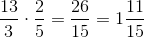# High School Math : Elementary Operations

## Example Questions

### Example Question #1 : Understanding Addition And Subtraction

Simplify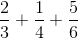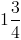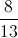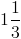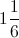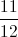Explanation:

Find the least common denominator (LCD) and convert each fraction to the LCD and then add.  Simplify as necessary.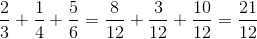The result is an improper fraction because the numerator is larger than the denominator and can be simplified and converted to a mix numeral.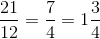### Example Question #1 : Mathematical Relationships And Basic Graphs

Simplify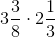.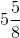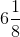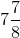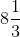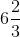Explanation:

Chenge the mixed numbers into improper fractions by multiplying the whole number by the denominator and adding the numerator to get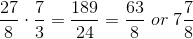.

### Example Question #2 : Mathematical Relationships And Basic Graphs

All of the following matrix products are defined EXCEPT: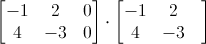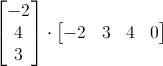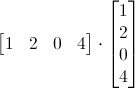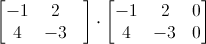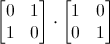Explanation:

Every matrix has a dimension, which is represented as the number of rows and columns. For example, a matrix with three rows and two columns is said to have dimension 3 x 2.

The matrix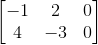has two rows and three columns, so its dimension is 2 x 3. (Remember that rows go from left to right, while columns run up and down.)

Matrix multiplication is defined only if the number of columns in the first matrix is equal to the number of rows on the second matrix. The easiest way to determine this is to write the dimension of each matrix. For example, let's say that one matrix has dimension a x b, and the second matrix has dimension c x d. We can only multiply the first matrix by the second matrix if the values of b and c are equal. It doesn't matter what the values of a and d are, as long as b (the number of columns in the first matrix) matches c (the number of rows in the second matrix).

Let's go back to the problem and analyze the choice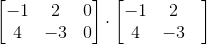.

The dimension of the first matrix is 2 x 3, because it has two rows and three columns. The second matrix has dimension 2 x 2, because it has two rows and two columns.

We can't multiply these matrices because the number of columns in the first matrix (3) is not equal to the number of rows in the second matrix (2). Thus, this product is not defined.

The answer is.

### Example Question #3 : Mathematical Relationships And Basic Graphs

Simplify.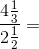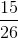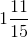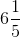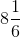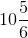Convert the mixed numbers into improper fractions by multiplying the whole number by the denominator and adding the numerator to get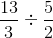Dividing by a fraction is the same as multiplying by its reciprocal so the problem becomes